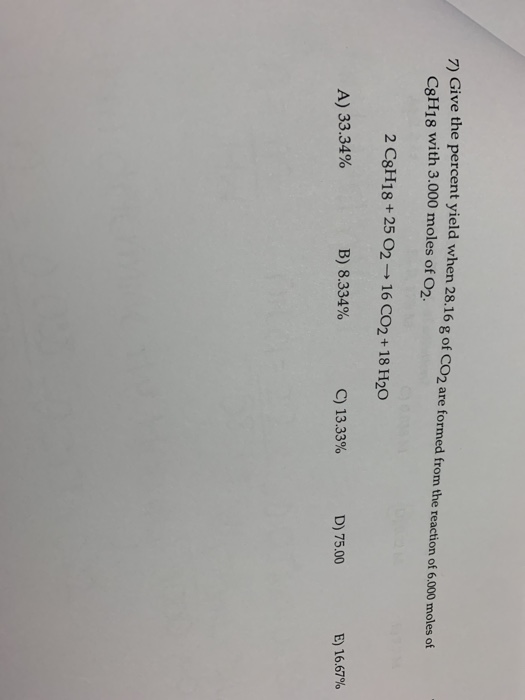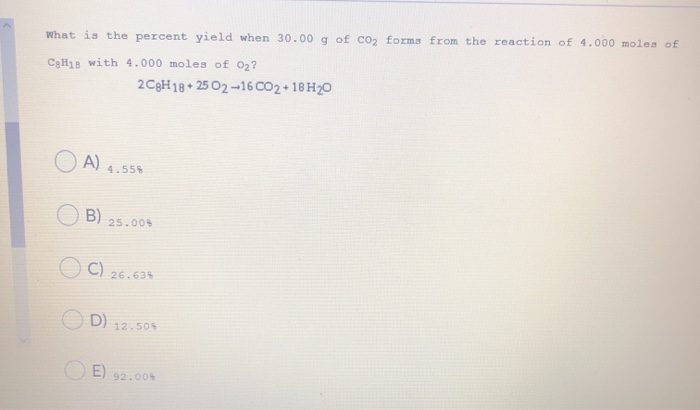# Give the percent yield when 28.16 g of CO2 are formed from the reaction of 8.000 moles of C8H18 with 4.000 moles of O2. 2 C8H18 + 25 O2 → 16 CO2 + 18 H2O

This Homework Help Question: "percent yelid" No answers yet.

We need 10 more requests to produce the answer to this homework help question. Share with your friends to get the answer faster!

0 /10 have requested the answer to this homework help question.

Once 10 people have made a request, the answer to this question will be available in 1-2 days.
All students who have requested the answer will be notified once they are available.
Similar Homework Help Questions
• ### Give the percent yield when 28.16 g of CO2 are formed from the reaction of 4.000 moles of C8H18...

Give the percent yield when 28.16 g of CO2 are formed from the reaction of 4.000 moles of C8H18 with 8.000 moles of O2.2 C8H18 + 25 O2 ? 16 CO2 + 18 H2Oa)12.50%b)25.00%c)20.00%d)50.00%

• ### Give the percent yield when 28.16 g of CO2 are formed from the reaction of 4.000...

Give the percent yield when 28.16 g of CO2 are formed from the reaction of 4.000 moles of C8H18 with 4.000 moles of O2. 2 C8H18 + 25 O2 → 16 CO2 + 18 H2O 20.00% 50.00% 25.00% 12.50%

• ### 12) G ive the percent yield when 28.16 g of CO2 are formed from the reaction of 4.000 moles of...

12) G ive the percent yield when 28.16 g of CO2 are formed from the reaction of 4.000 moles ofC8H18 with 4.000 moles of O2.2 C8H18 + 25 O2 ? 16 CO2 + 18 H2O

• ### Percent Yield

Give the percent yield when 28.16 g of CO2 are formedfrom the reaction of 4.000 moles of C8H18with 4.000 moles ofO2.2 C8H18 + 25 O2 → 16CO2 + 18 H2OA.25.00%B.89.90%C.22.0%D.20.00%

• ### 7) Give the percent yield when 28.16 g of CO2 are formed from the reaction of...7) Give the percent yield when 28.16 g of CO2 are formed from the reaction of 6.000 moles of C8H18 with 3.000 moles of 02 2 C8H18+25 02 16 CO2+ 18 H20 A) 33.34% B) 8.334% C) 13.33% D) 75.00 E) 16.67%

• ### 2 C8H18 (l)    +    25 O2 (g) ---->   16 CO2 (g)    +     18 H2O (g) (OR...

2 C8H18 (l)    +    25 O2 (g) ---->   16 CO2 (g)    +     18 H2O (g) (OR 2 C8H18 (l)    +    25 O2 (g) right arrow   16 CO2 (g)    +     18 H2O (g)) Use the following conversions: 1 mol = MW in g (2 d.p.) = 22.414 L All gases are at STP.   How many liters of CO2 would be produced from the reaction of 5.0 L of C8H18 with 55 L of O2? Theoretical yield of CO2 from 5.0 L...

• ### The combustion of octane (C8H18) in oxygen proceeds as follows 2 C8H18(g) + 25 O2(g) --->...

The combustion of octane (C8H18) in oxygen proceeds as follows 2 C8H18(g) + 25 O2(g) ---> 16 CO2 (g) + 18 H2O(l) How many moles of CO2are produced when 5.0 moles of octane, C8H18, is burned in 5.0 moles of oxygen?

• ### What is the percent yield when 30.00 g of CO2 forms from the reaction of 4.000...What is the percent yield when 30.00 g of CO2 forms from the reaction of 4.000 moles of CH18 with 4.000 moles of 02? 2C9H 18+ 25 02-16CO2 +18 H20 O A) 4.558 OB) 25.005 OC) 26.633 D) 12.505 E) 92.003

• ### The complete combustion of octane

The complete combustion of octane, C8H18, the main componentof gasoline, proceeds as follows:2 C8H18(l) + 25 O2(g)----->16 CO2(g) + 18 H2O(g)(a) How many moles of O2 are needed to burn 1.50 mol ofC8H18?(b) How many grams of O2 are needed to burn 10.0 g ofC8H18?please show all work

• ### This reaction shows the complete combustion of octane, C8H18, a component of gasoline....?

2 C8H18(l) + 25 O2(g) 16 CO2(g) + 18 H2O(l) (a) How many moles of O2 are needed to burn 2.10 mol of C8H18? (b) How many grams of NaF form when 0.800 mol of HF reacts in this way? (c) How many grams of Na2SiO3 can be dissolved by 0.600 g of HF?

Free Homework App